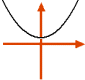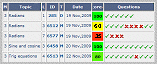Go to content
Which equation might have the graph shown here?It is used on o-test pages, where you can repeat the test as often as you like and if you are logged in using a personal ID your score will be saved. You can view all your saved scores by clicking on your user name and following the links there. For more information on this see Help on assessment

## Glossary

### equation

A statement that two mathematical expressions are equal.

### graph

A diagram showing a relationship between two variables.
The diagram shows a vertical y axis and a horizontal x axis.

Full Glossary List

## This question appears in the following syllabi:

SyllabusModuleSectionTopicExam Year
AP Calculus AB (USA)2Algebra and FunctionsQuadratic curves-
AP Calculus BC (USA)2Algebra and FunctionsQuadratic curves-
AQA A-Level (UK - Pre-2017)C1Algebra and FunctionsQuadratic curves-
AQA AS Maths 2017Pure MathsAlgebraQuadratic Curves-
AQA AS/A2 Maths 2017Pure MathsAlgebraQuadratic Curves-
CBSE IX (India)AlgebraPolynomialsConstant, linear, quadratic and cubic-
CCEA A-Level (NI)C1Algebra and FunctionsQuadratic curves-
CIE A-Level (UK)P1Algebra and FunctionsQuadratic curves-
Edexcel A-Level (UK - Pre-2017)C1Algebra and FunctionsQuadratic curves-